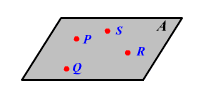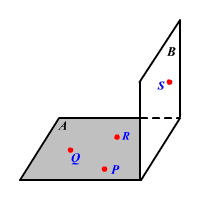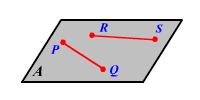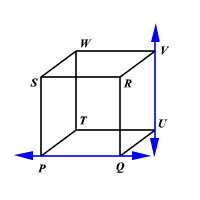# Coplanar

Points or lines are said to be coplanar if they lie in the same plane.

Example 1:The points $P$ , $Q$ , and $R$ lie in the same plane $A$ .

They are coplanar .

Example 2:The points $P$ , $Q$ and $R$ lie in the plane $A$ and the point $S$ lies on the plane $B$ .

They are non coplanar .

Example 3:The two lines $\stackrel{↔}{PQ}$ and $\stackrel{↔}{RS}$ lie in the same plane $A$ .

They are coplanar .

Example 4:The two lines $\stackrel{↔}{PQ}$ and $\stackrel{↔}{UV}$ are skew lines.

They are non coplanar .